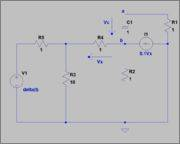# RC Impulse Response

Hello. I would need some clarifications about the impulse response of a RC circuit.

1. Homework Statement

Find the impulse response relative to the vc(t) voltage.NOTE: delta(t) is the exact dirac's delta of infinite amplitude, not an approximated peak.

## Homework Equations

δ(t) = d θ(t) / dt

## The Attempt at a Solution

First of all I have a doubt of theoretical nature. If we know that capacitor's voltage is a continuous function (vc(0-) = vc(0+), and know that vc = 0 for t < 0, how can voltage change? Shouldn't it remain 0 for the impulse duration, considering the circuit completely ideal?

Anyway, is it correct to consider the impulse source as a step one, and then derivate the function?

Specifically talking, I found the Thevenin equivalent between a and b, and it comes out:

Rth = 1,6344 Ω
Vth = -0.3313 V

Then I calculate the step response as:

vcθ(t) = -0.3323 θ(t) (1 - e-t/1.6344)

From here is it correct to just derivate this function to get the impulse response?

vcδ(t) = -0.3323/1.6344 e-t/1.6344

Thank you very much.

gneill
Mentor
That works. Is it possible that you've got the sign wrong? My interpretation of the voltage labeling (arrows) on the schematic would make the node b end of the capacitor the positive reference for the capacitor voltage, and the negative reference for Vx. In other words, Vc = Vb - Va. But the numerical constants look right to me.

You could also solve the problem using Laplace Transforms. The transform of the dirac impulse is simply "1".

Oh, your thread title is misleading: There's no "L" in the circuit, so "RL Impulse Response" doesn't match the problem given.

•Frank-95
That works. Is it possible that you've got the sign wrong?

Yes I probably mistakened the sign.

You could also solve the problem using Laplace Transforms. The transform of the dirac impulse is simply "1".

Unluckily the university course does not consider laplace transform as a way to solve circuits.

Oh, your thread title is misleading: There's no "L" in the circuit, so "RL Impulse Response" doesn't match the problem given.

I apologise. Basically I had written RL, then I noticed the error and wrote RC, but the log in expired and when I refreshed the page there was RL again, but I couldn't change the title once the thread was opened.

Thank you very much.

gneill
Mentor
Yes I probably mistakened the sign.
No worries. It happens.
Unluckily the university course does not consider laplace transform as a way to solve circuits.
Wow. Laplace transforms must be one of the most powerful methods for handling differential equations. They're used all the time in circuit analysis. That's a bit of a handicap for you.
I apologise. Basically I had written RL, then I noticed the error and wrote RC, but the log in expired and when I refreshed the page there was RL again, but I couldn't change the title once the thread was opened.
No problem, I can fix it for you.